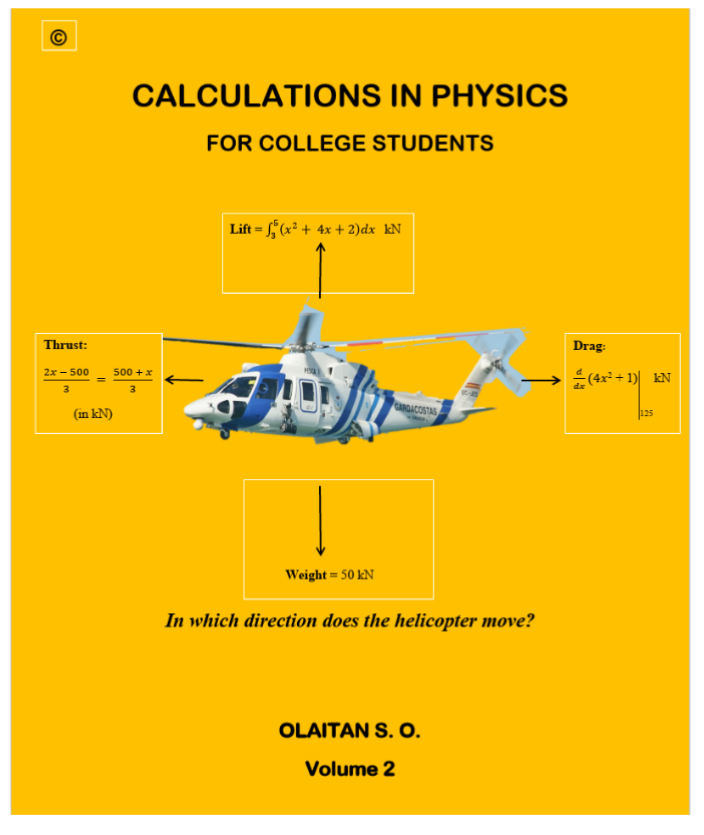# “CALCULATIONS IN PHYSICS FOR COLLEGE STUDENTS – VOLUME 2” – A NEW PHYSICS TEXTBOOK BY OLAITAN S. O.The book covers calculations in WAEC’s current senior secondary school Physics curriculum, calculations in all JAMB’s topics required for UTME, calculations in some IGCSE topics, calculations in NECO’s entire current Physics curriculum and calculations in few Cambridge AS Level topics.

All the relevant formulas needed for WAEC’s Physics curriculum, NECO’s Physics curriculum, JAMB’s Physics curriculum for UTME and IGCSE Physics curriculum are included in the book.

This Volume 2 of the book contains calculations in the following topics: Machines, Pressure in Fluids, Waves and Applications, Sound Waves, Linear, Area and Cubic Expansivities, Temperature and Its Measurement, Measurement of Heat Energy, Current Electricity and Capacitors.

The book is mainly for SS1 (Year 10) to SS3 (Year 12) senior secondary students.

In every topic in each chapter of the book, all possible calculations a student is likely to come across are solved as examples with detailed explanations. Some of the solved calculations are actually past Physics questions from WAEC, NECO, JAMB, IGCSE and Cambridge AS Level.

Immediately after the solved calculations, you would find exercise containing several practice questions for students to practice. These practice questions are majorly from WAEC, NECO, JAMB and IGCSE. Students can later check the answers to the practice questions on the answer page.

This book will greatly help the students solve calculations in Physics.

For enquiries, contact: (+234)08033487161 or (+234)08177093682 or osospecial2015@yahoo.com

For Abuja residents, you can get the copy of the book from this contact: 08034845843

Check the preview of the book below:

Related:

“CALCULATIONS IN PHYSICS FOR COLLEGE STUDENTS – VOLUME 3” – A NEW PHYSICS TEXTBOOK BY OLAITAN S. O.